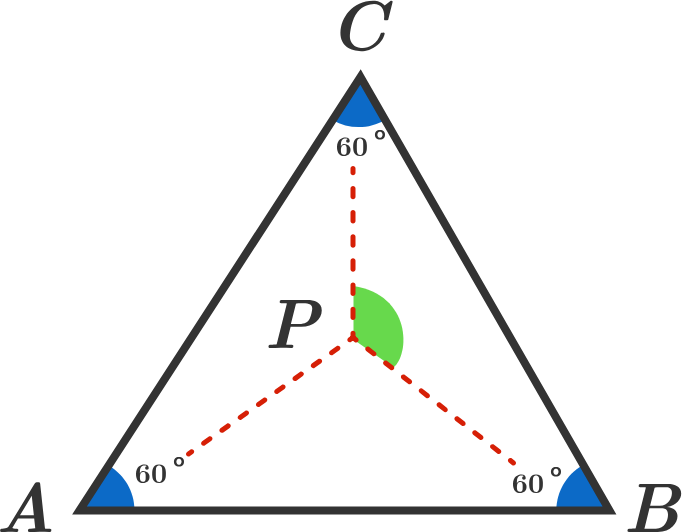Equilateral Triangle ChallengeGiven that $\triangle ABC$ is an equilateral triangle, with a point $P$ inside of it such that

$PA^2 =PB^2 + PC^2,$

find the measure of $\angle BPC$ in degrees.

×